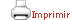Teaching plan for the course unit

 Català English CloseGeneral information

Course unit name: Mathematical and Statistical Techniques

Course unit code: 568423

Coordinator: Francesc Xavier Luri Carrascoso

Department: Department of Quantum Physics and Astrophysics

Credits: 6

Single program: S

 Estimated learning time Total number of hours 150

 Face-to-face and/or online activities 60
 -  Lecture Face-to-face 60
 Supervised project 40
 Independent learning 50

 Recommendations

 Students are recommended to have basic programming skills to follow this course.

 Competences to be gained during study

 — Understand a problem, connect it with acquired knowledge, propose a solution and test it. — Follow a course taught in English. — Follow (slightly) abstract arguments leading to fundamental theorems, understand their meaning and how to use them, e.g. in a physical context. — Acquire basic programming skills and usage of basic tools for the handling of data (generation of graphics and handling of data files). — Become familiar with basic calculus.

 Learning objectives
 Referring to knowledge — Get acquainted with fundamental results of probability theory and statistics. Understand their relevance to important issues in experimental and theoretical physics. — Understand the power and limitations of Monte-Carlo methods, in particular when applied to physical contexts, such as quantum field theory. — Develop comprehensive skills on the topic, ranging from the ability to use software (such as R) to perform computations on specific data to the ability to prove easy mathematical statements in order to solve theoretical issues. — Get acquainted with the techniques for data analysis and the basic concepts of data mining.

 Teaching blocks

1. Fundamental concepts of probability theory and statistics

*  General review of probability theory: random variables and events; Bayes’ theorem; Probability distributions: binomial, Poisson, Gaussian, Cauchy; Law of large numbers; Hoeffding’s inequality; Central-limit theorem and Berry-Esseen theorem

* Introduction to R

* Statistical inference: point estimation theory, confidence intervals, chi-squared test, Fisher information, Cramer-Rao bound, maximum-likelihood estimators, hypothesis testing, Kolmogorov-Smirnov tests, least squares, information theory (typicality, mutual information, correlations)

2. Monte Carlo methods

*  Generalities; Sampling, integration, optimisation

* Importance sampling, stratified sampling, rejection sampling

* Metropolis algorithm; Generalities: reversibility, strong ergodicity and convergence; A priori probabilities, parallel tempering, simulated annealing

* Applications of the Metropolis algorithm to statistical physics, quantum field theory and events generation

3. Multivariate analysis and statistical treatment techniques

*  Data analysis and representation; Statistical distances; Principal component analysis
* Hierarchical and non-hierarchical clustering
* Discriminant analysis
* Neural networks
* Support vector machines
* Non-parametric methods of estimation of a probability density function: histograms, simple estimators, kernel estimators

4. Databases and data mining

*  Basic concepts
* Introduction to data mining
* Case study: the Gaia archive

5. Practical work

*  Introduction to R
* Introduction to WEKA (data analysis and data mining)

 Official assessment of learning outcomes

 There is no exam for this subject. Instead, 5 problem-solving assignments are set during the course. Grading is based on the assessment of the reports submitted. Repeat assessment: students have to repeat and resubmit the 5 problem-solving assignments following the instructions from the lecturers. Once the assignments have been assessed, students take an oral exam on their contents. If this exam is successfully passed, the final grade is calculated from the marks of the assignments; otherwise, the subject is graded as failed.   Examination-based assessment